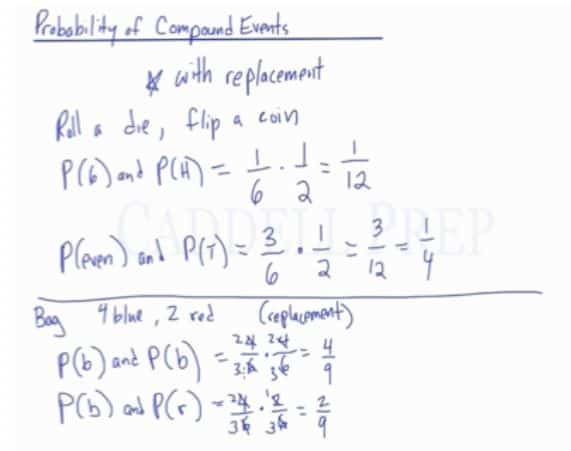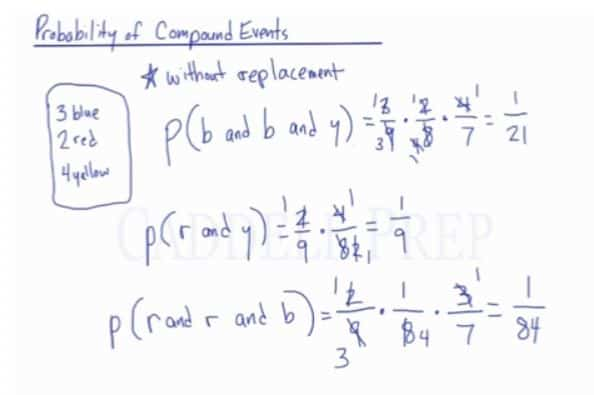The Probability of Compound Events with Replacement

In the first video, we are going to work with the probability of compound events. After you finish this lesson, view all of our Algebra 1 lessons and practice problems.

The probability of Compound Events: The probability of multiple events happening

For rolling a dice and flipping a coin:$P(6)$ and$P(Head)$
The probability of rolling a dice is$\frac{1}{6}$ and the probability of flipping a head is$\frac{1}{2}$

Multiplying the two probability together, since both events have to be happening, we now have$\frac{1}{6}\times\frac{1}{2}=\frac{1}{12}$

Examples of Probability Of Compound Events with Replacement

Example 1

Spin a spinner numbered$1-8$, and you land on$8$ twice in a row

The probability number$8$ will land is$\dfrac{1}{8}$

Since it landed twice in row, we have:$P(8)=\dfrac{1}{8}\times \dfrac{1}{8} = \dfrac{1}{64}$

Example 2

Rolling a die and getting a number other than$4$ twice in a row

The probability of rolling a dice other than$4$ is$\frac{5}{6}$

Since the numbers other than 4 appeared two times, we have:$P(1,2,3,5 and 6)=\dfrac{5}{6}\times \dfrac{5}{6} = \dfrac{25}{36}$

Video-Lesson Transcript

In this lesson, we’ll review the probability of compound events with replacement.

Don’t worry what this means, it will make more sense afterward.

For example, we want to roll a die and flip a coin.

We want to know the probability of rolling a$6$ and getting heads.$P(6) and P(H)$

When we have a compound event, we can just actually multiply the probabilities.$P(6) and P(H)$$P(6) and P(H) = \dfrac{1}{6} \times \dfrac{1}{2} = \dfrac{1}{12}$

The probability of both of them occurring at the same time is$\dfrac{1}{12}$.

Let’s get the probability of rolling an even number and getting a tail.$P(even) and P(T)$

Out of six numbers on a die, three of them are even.$P(6) and P(T) = \dfrac{3}{6} \times \dfrac{1}{2} = \dfrac{3}{12} = \dfrac{1}{4}$

The probability of getting an even number and flipping tails is one out of every four flips.

Let’s look at a different example now.

You have a bag with$4$ blue marbles and$2$ red marbles.

What’s the probability of getting a blue marble and replacing it and then picking another blue marble?

Replacing it means putting it in the bag again.$P(b) and P(b) = \dfrac{4}{6} \times \dfrac{4}{6} = \dfrac{2}{3} \times \dfrac{2}{3} = \dfrac{4}{9}$

Let’s look at another one.

The probability of getting a blue marble and after you pick it, you put it back in the bag and then afterward picking a red marble.$P(b) and P(r) = \dfrac{4}{6} \times \dfrac{2}{6} = \dfrac{2}{3} \times \dfrac{1}{3} = \dfrac{2}{9}$This is an example of finding the probability with replacement.

If we take something out and we put it back in.

The denominator stays the same because you start off with six marbles and when you put the marble you picked back in, six marbles are still there. The probability of the second outcome is still with six marbles.

The Probability Of Compound Events Without Replacement

In the second video, we are going to work with the probability of compound events without replacement.

Without replacement, the total number of outcomes decreases after each drawing.

For example:
In a bag, there are 3 blue marbles, 2 red marbles, and 4 yellow marbles. What is the probability of drawing two blue marbles and one yellow marble without placing the marbles back into the bag?

Here, we would have$P(blue, blue, yellow)$

Which is the same thing as$P(blue, blue, yellow)=\frac{3}{9}\times\frac{2}{8}\times\frac{4}{7}$
after substitution

Reduce any term if necessary$P(blue, blue, yellow)=\frac{1}{3}\times\frac{1}{4}\times\frac{4}{7}$$P(blue, blue, yellow)=\frac{1}{3}\times\frac{1}{1}\times\frac{1}{7}$

Multiply across$P(blue, blue, yellow)=\frac{1}{21}$

Examples of Probability Of Compound Events without Replacement

Example 1

I choose$2$ balls at random from a bag containing$7$ blue and$5$ red balls. Find the probability of getting$2$ blue balls if I don’t replace the first ball before selecting the second

Without replacement, the total number of outcomes decreases after each drawing.

We have:$P(BB)=\dfrac{7}{12}\times\dfrac{6}{11}$$P(BB)=\dfrac{7}{22}$

Example 2

A packet of sweets has$3$ pink,$2$ green and$5$ blue sweets. a green and a pink sweet are removed from the packet. One at a time, without replacement.

Without replacement, the total number of outcomes decreases after each drawing.

We have:$P(GP)=\dfrac{3}{10}\times\dfrac{2}{9}$$P(GP)=\dfrac{2}{15}$

Therefore, the probability of drawing two blue marbles and a yellow marble without replacement would be$\frac{1}{21}$.

Video-Lesson Transcript

In this lesson, we’ll look into the probability of compound events but this time without replacement.

For example, we’re taking marbles out of a jar, we won’t replace it next time.

So let’s look at an example here.

We have a jar that contains$3$ blue marbles,$2$ red marbles, and$4$ yellow marbles.

We want to know what is the probability of getting a blue marble and a second blue marble, and then a yellow marble.$P(blue, blue, yellow)$

We’re going to multiply these probabilities together to find the final probability.

But we’re not replacing the marble after we take it out.

Let’s see how many marbles we have to begin with.

We have$3 + 2 + 4 = 9$.

So, we have$9$ marbles in the beginning.

Initially, the probability of getting a blue marble is$3$ out of$9$.

Now, we want to see the probability of the second one being blue.

After we drew one marble, there is one less blue marble in the jar. So, there are just two blue marbles to choose and one less marble altogether. So now, there are only eight marbles left. Which is$2$ out of$8$.

And now, we want to draw a yellow marble. There are still$4$ yellow marbles in the jar. But at this point, since we took$2$ marbles out, we just have$7$ marbles left altogether.

Then, let’s multiply these together.$P(blue, blue, yellow) = \dfrac{3}{9} \times \dfrac{2}{8} \times \dfrac{4}{7} = \dfrac{1}{3} \times \dfrac{2}{8} \times \dfrac{4}{7} = \dfrac{1}{21}$

So, the odds of selecting a blue marble followed by another blue marble and then a yellow marble is$\dfrac{1}{21}$.

What is the probability of drawing a red marble then a yellow marble?$P(red, yellow)$

The probability of selecting a red would be$2$ out of$9$.

Because there are$9$ marbles in the jar.

After we select it, we do not replace it. So now, there’s only$8$ marbles total.

There are still$4$ yellow marbles in a total of$8$.$P(red, yellow) = \dfrac{2}{9} \times \dfrac{4}{8} = \dfrac{2}{9} \times \dfrac{1}{2} = \dfrac{1}{9}$

One more example.

We’ll do the probability of selecting a red marble and another red marble and a blue marble.$P(red, red, blue)$

Initially, there are$9$ marbles.

So, the probability of selecting a red marble is$2$ out of$9$.

Now, there’s one less red marble and one less marble altogether.

So, we’re left with$1$ red marble out of only$8$.

The probability of selecting a blue is still$3$ marbles out of$7$ marbles left. Because we already took out$2$ earlier.$P(red, red, blue) = \dfrac{2}{9} \times \dfrac{1}{8} \times \dfrac{3}{7} = \dfrac{1}{84}$

So, the probability of getting a red then another red and then a blue is$\dfrac{1}{84}$.This is the probability of compound events without replacement.

Once you select a marble, you remove it so you’ll have one less from the total.